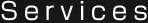# Moment of Inertia J Calculation Formula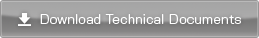### Moment of Inertia Calculation Formula

#### Moment of Inertia of a Hollow CylinderD : Outer diameter of the cylinder[m]
d : Inner diameter of the cylinder[m]
M : Mass of the cylinder[kg]

 J= 1 M (D2 + d2 ) [kg･m2 ] 8

#### Moment of Inertial of a Cylinder with a Displaced Center-of-Rotation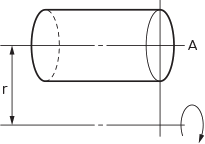M : Mass of the cylinder[kg]
J A : Moment of inertia of the cylinder rotating around r the center axis A [kg・m 2 ]

 J=JA + M･r2 [kg･m2]

#### Moment of Inertia of a Rotating Rod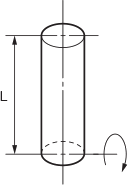L : Length of the rod[m]
M : Mass of the rod[kg]

 J= 1 M･L2 [kg･m2 ] 3

#### Moment of Inertial of a Rod with a Displaced Center-of-Rotation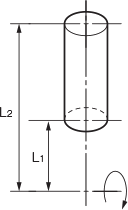L1, L2 : Distance from the center of rotation [m]
M : Mass of the rod[kg]

 J= 1 M(L12 + L1L2 + L22) [kg･m2 ] 3

#### Moment of Inertia of the Winding Mechanism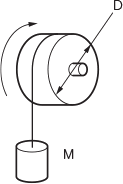JA : Moment of inertia of the drum[kg・m2]
D : Diameter of the drum[m]
M : Mass of the load[kg]

 J=JA + 1 M･D2 [kg･m2 ] 4

#### Moment of Inertia with CounterbalanceJA : Moment of inertia of the drum[kg・m2]
D: Diameter of the drum[m]
M 1,M2 : Mass[kg]

 J=JA + 1 (M1+M2) D2 [kg･m2 ] 4

#### Moment of Inertia of a Cuboid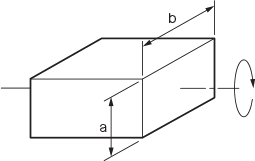a, b : Side length[m]
M : Mass of the cuboid[kg]

 J= 1 M(a2 + b2)[kg・m2 ] 12

#### Moment of Inertia of an Object that Moves in a Straight Line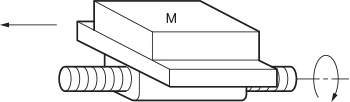JA : Moment of inertia of the feed screw[kg・m2]
M : Mass of the load[kg]

 J=JA + M･P2 [kg･m2 ] 4π2

#### Moment of Inertia of the Rack and Pinion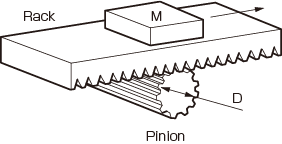JA : Moment of inertia of thepinion[kg・m2]
D : Diameter of the pinion[m]
M : Mass of the rack and load[kg]

 J=JA + 1 M･D2 [kg･m2 ] 4

#### Moment of Inertia of the Belt Conveyor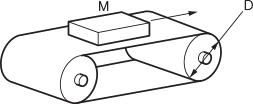JA : Moment of inertia of the roller[kg・m2]
D : Diameter of the roller[m]
M : Mass of the load[kg]

 J=JA + 1 M･D2 [kg･m2 ] 4

#### Moment of Inertia when a Workpiece Is Held by the Rollers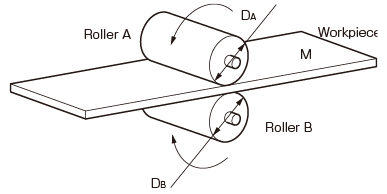JA : Moment of inertia of the roller A[kg・m2]
J B : Moment of inertia of the roller B[kg・m2]
D A : Diameter of the roller[m]
D B : Diameter of the roller B[m]
M: Equivalent mass of the workpiece[kg]

 J=JA+ ( DA )2JB + 1 M･DA2 [kg・m2 ] DB 4

#### Motor Shaft Conversion Moment of InertiaZ1: Teeth number of the motor-side gear
Z 2 : Teeth number of the load-side gear
R : Speed reduction ratio Z2/Z1
J A : Moment of inertia of load[kg・m2]
J 1 : Moment of inertia of the motor-side gear[kg・m2]
J 2 : Moment of inertia of the load-side gear[kg・m2]

 J=J1 +(JA + J2)･( 1 )2 [kg･m2 ] R

Other Technical Documents

﻿